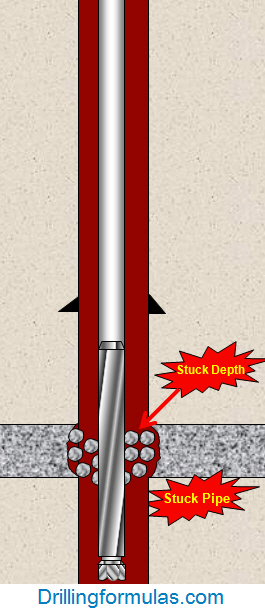## Determine how many feet of free pipe without free pipe constant table

You may sometimes find the table for Free Point Constant for your stuck pipe calculation so you use the formula from the previous post to determine Free Point Constant and apply it into free pipe formula to determine where the depth of stuck pipe is.Stuck Pipe Calculation – Stuck Depth

The steps of calculation are as follows:

1 ) Determine the free point constant (FPC) by this following formula:

FPC = As x 2500

where: As = pipe wall cross sectional area, sq in.

As = (OD2-ID2) x 0.7854

OD and ID are inch unit

2) Determine depth of stuck pipe by this following formula:

Depth of stuck pipe = (Pipe stretch in inch x free point constant (FPC)) ÷ Pull force in thousands of pounds

Please see the example below to demonstrate you how to apply those 2 formulas above into real drilling operation.

Example#2: Determine the free point constant (FPC) and the depth the pipe is stuck using the following information:

3-1/2” tubing # 9.5 lb/ft

3-1/2” tubing ID = 2.992 inch

20 inch of stretch with 25 Klb of pulling force

a) Determine free point constant (FPC):

FPC = (3.52 – 2.9922) x 0.7854 x 2500

FPC = 6475.5

b) Determine the depth of stuck pipe:

Feet of free pipe (ft) = (20 inch x 6475.5) ÷ 25

** 25 is the kilo-force to pull to 20 inch **

Feet of free pipe (ft) = 5,180 ft

Please find the Excel sheet used for calculating depth of stuck pipe.